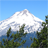# Nested if statements

327
3
Jump to solution
09-25-2018 06:19 AMby
New Contributor III

I am trying to assign a label based on a size range but it is not working. Here is the stament I am using.

if(\${c_lenght_cal} <= 5, "Hatchling", (if\${c_lenght_cal} >5 and \${c_lenght_cal} <=10, "Post-hatchling",(if\${c_lenght_cal} >10 and \${c_lenght_cal} <=60, "Juvenile", (if\${c_lenght_cal} >60 and \${c_lenght_cal} <=92, "Subadult", "Adult"))))

What am I missing?

Thanks

1 Solution

Accepted Solutionsby
Occasional Contributor II

It looks like you have the parentheses within the statement on the wrong side of the ifs.

This worked for me:

if(\${c_length_cal} <= 5, "Hatchling", if(\${c_length_cal} > 5 and \${c_length_cal} <= 10, "Post-hatchling", if(\${c_length_cal} > 10 and \${c_length_cal} <=60, "Juvenile", if(\${c_length_cal} > 60 and \${c_length_cal} <= 92, "Subadult","Adult"))))

For what it's worth, I think you might be able to shorten this statement as well. I don't think you need the > statements, since the previous <= statement will have already evaluated to true.

if(\${c_length_cal} <= 5, "Hatchling", if(\${c_length_cal} <= 10, "Post-hatchling", if(\${c_length_cal} <=60, "Juvenile", if(\${c_length_cal} <= 92, "Subadult","Adult"))))

3 Repliesby
Occasional Contributor II

It looks like you have the parentheses within the statement on the wrong side of the ifs.

This worked for me:

if(\${c_length_cal} <= 5, "Hatchling", if(\${c_length_cal} > 5 and \${c_length_cal} <= 10, "Post-hatchling", if(\${c_length_cal} > 10 and \${c_length_cal} <=60, "Juvenile", if(\${c_length_cal} > 60 and \${c_length_cal} <= 92, "Subadult","Adult"))))

For what it's worth, I think you might be able to shorten this statement as well. I don't think you need the > statements, since the previous <= statement will have already evaluated to true.

if(\${c_length_cal} <= 5, "Hatchling", if(\${c_length_cal} <= 10, "Post-hatchling", if(\${c_length_cal} <=60, "Juvenile", if(\${c_length_cal} <= 92, "Subadult","Adult"))))by
New Contributor III

Stephen

Thanks I just figure out the parentheses and got it working a little while ago also.

I like the idea of shortening the statement so I will give that a try !byMVP Esteemed Contributor

If Stephen's suggestions work for you, please mark his comment as the answer to close out this question.